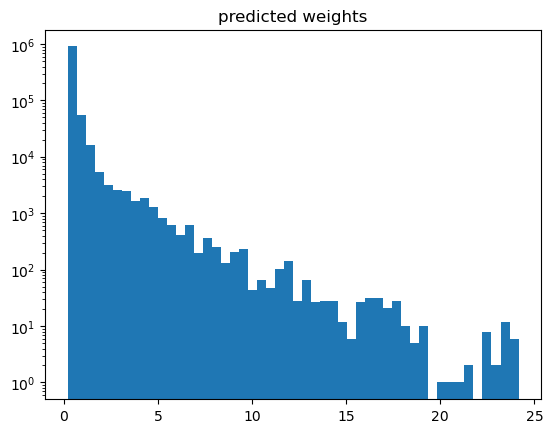# 7: Demonstration of distribution reweighting

• Reweighting in HEP is used to minimize difference between real data and Monte-Carlo simulation.

• Known process is used, for which real data can be obtained.

• Target of reweighting: assign weights to MC such that MC and real data distributions coincide.

hep_ml.reweight contains methods to reweight distributions. Typically we use reweighting of monte-carlo to fight drawbacks of simulation, though there are many applications.

In this example we reweight multidimensional distibutions: original and target, the aim is to find new weights for original distribution, such that these multidimensional distributions will coincide.

Here we have a toy example without a real physics meaning.

Pay attention: equality of distibutions for each feature $$\neq$$ equality of multivariate distributions.

All samples are divided into training and validation part. Training part is used to fit reweighting rule and test part is used to estimate reweighting quality.

:

%matplotlib inline

import numpy as np
import pandas as pd
import uproot
from hep_ml import reweight
from matplotlib import pyplot as plt


:

columns = ['hSPD', 'pt_b', 'pt_phi', 'vchi2_b', 'mu_pt_sum']

httpsource={'chunkbytes': 1024*1024, 'limitbytes': 33554432, 'parallel': 64}
) as original_file:
original_tree = original_file['tree']
original = original_tree.arrays(library='pd')
httpsource={'chunkbytes': 1024*1024, 'limitbytes': 33554432, 'parallel': 64}
) as target_file:
target_tree = target_file['tree']
target = target_tree.arrays(library='pd')
original_weights = np.ones(len(original))


## prepare train and test samples

• train part is used to train reweighting rule

• test part is used to evaluate reweighting rule comparing the following things:

• Kolmogorov-Smirnov distances for 1d projections

• n-dim distibutions using ML (see below).

:

from sklearn.model_selection import train_test_split

# divide original samples into training ant test parts
original_train, original_test = train_test_split(original)
# divide target samples into training ant test parts
target_train, target_test = train_test_split(target)

original_weights_train = np.ones(len(original_train))
original_weights_test = np.ones(len(original_test))

:

from hep_ml.metrics_utils import ks_2samp_weighted

hist_settings = {'bins': 100, 'density': True, 'alpha': 0.7}

def draw_distributions(original, target, new_original_weights):
plt.figure(figsize=[15, 7])
for id, column in enumerate(columns, 1):
xlim = np.percentile(np.hstack([target[column]]), [0.01, 99.99])
plt.subplot(2, 3, id)
plt.hist(original[column], weights=new_original_weights, range=xlim, **hist_settings)
plt.hist(target[column], range=xlim, **hist_settings)
plt.title(column)
print('KS over ', column, ' = ', ks_2samp_weighted(original[column], target[column],
weights1=new_original_weights, weights2=np.ones(len(target), dtype=float)))


### Original distributions

KS = Kolmogorov-Smirnov distance: a measure of how well two distributions agree, the lower the distance, the better the agreement. In this case we want a low KS value.

:

# pay attention, actually we have very few data
len(original), len(target)

:

(1000000, 21441)

:

draw_distributions(original, target, original_weights)

KS over  hSPD  =  0.5203540728277889
KS over  pt_b  =  0.21639364439970188
KS over  pt_phi  =  0.4020113592414034
KS over  vchi2_b  =  0.40466385087324064
KS over  mu_pt_sum  =  0.21639364439970188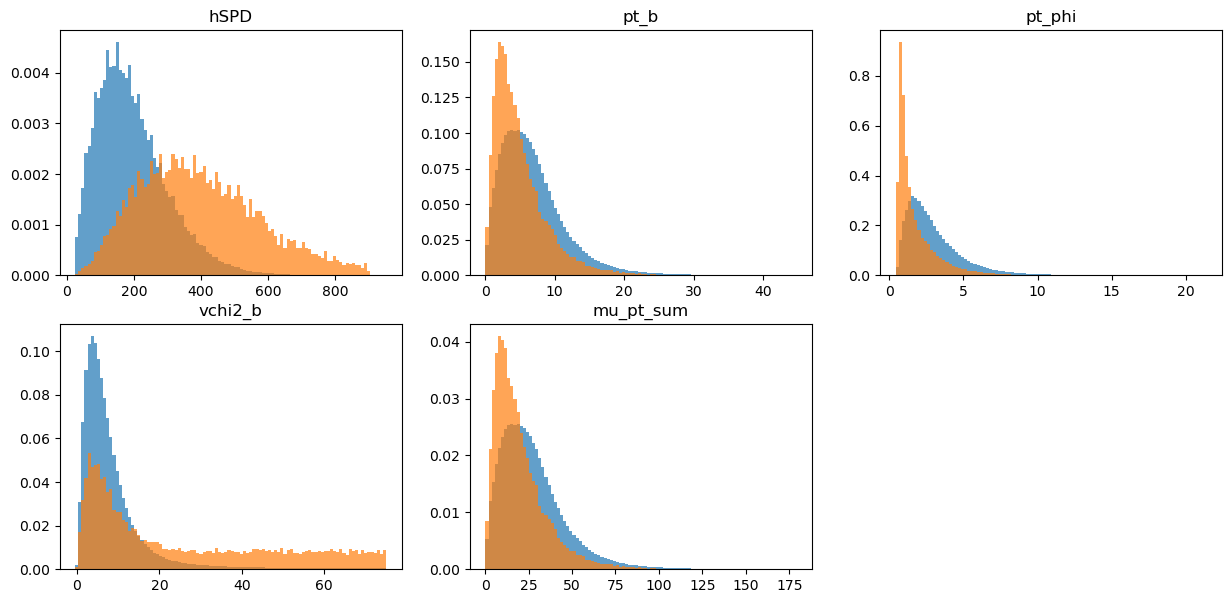## train part of original distribution

:

draw_distributions(original_train, target_train, original_weights_train)

KS over  hSPD  =  0.5170665174129254
KS over  pt_b  =  0.21549674626683318
KS over  pt_phi  =  0.40070777114440437
KS over  vchi2_b  =  0.40245931342664004
KS over  mu_pt_sum  =  0.21549674626683318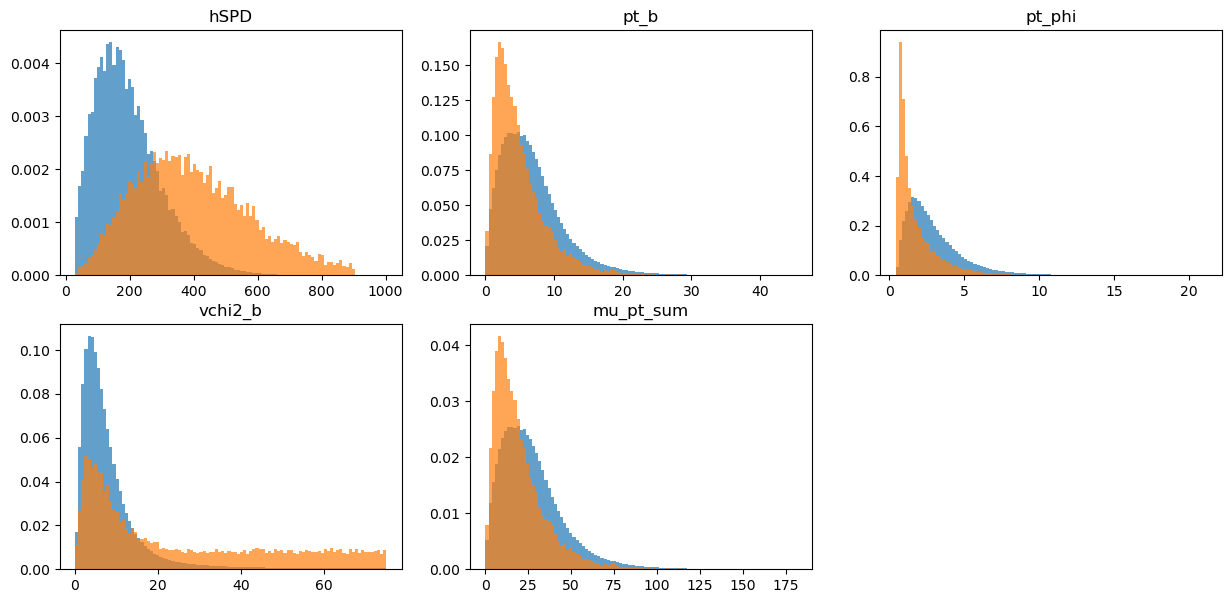## test part for target distribution

:

draw_distributions(original_test, target_test, original_weights_test)

KS over  hSPD  =  0.5334475269539376
KS over  pt_b  =  0.22120587987286633
KS over  pt_phi  =  0.40705874239868967
KS over  vchi2_b  =  0.4142118683089644
KS over  mu_pt_sum  =  0.22120587987286633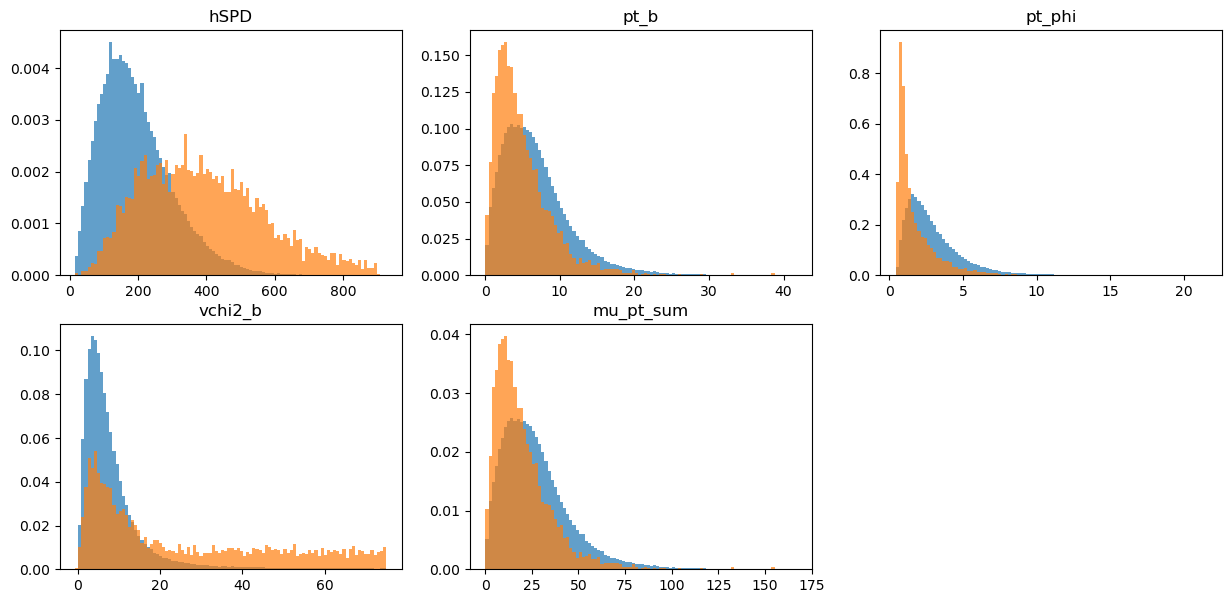## Bins-based reweighting in n dimensions

Typical way to reweight distributions is based on bins.

Usually histogram reweighting is used, in each bin the weight of original distribution is multiplied by:

$$m_{bin} = \frac{w_{target}}{w_{original}}$$

where $$w_{target}$$ and $$w_{original}$$ are the total weight of events in each bin for target and original distributions.

1. Simple and fast!

2. Very few (typically, one or two) variables

3. Reweighting one variable may bring disagreement in others

4. Which variable to use in reweighting?

:

bins_reweighter = reweight.BinsReweighter(n_bins=20, n_neighs=1.)
bins_reweighter.fit(original_train, target_train)

bins_weights_test = bins_reweighter.predict_weights(original_test)
# validate reweighting rule on the test part comparing 1d projections
draw_distributions(original_test, target_test, bins_weights_test)

KS over  hSPD  =  0.42863624879323187
KS over  pt_b  =  0.12643137585836178
KS over  pt_phi  =  0.2879532533863654
KS over  vchi2_b  =  0.35373773290850885
KS over  mu_pt_sum  =  0.12643137585836178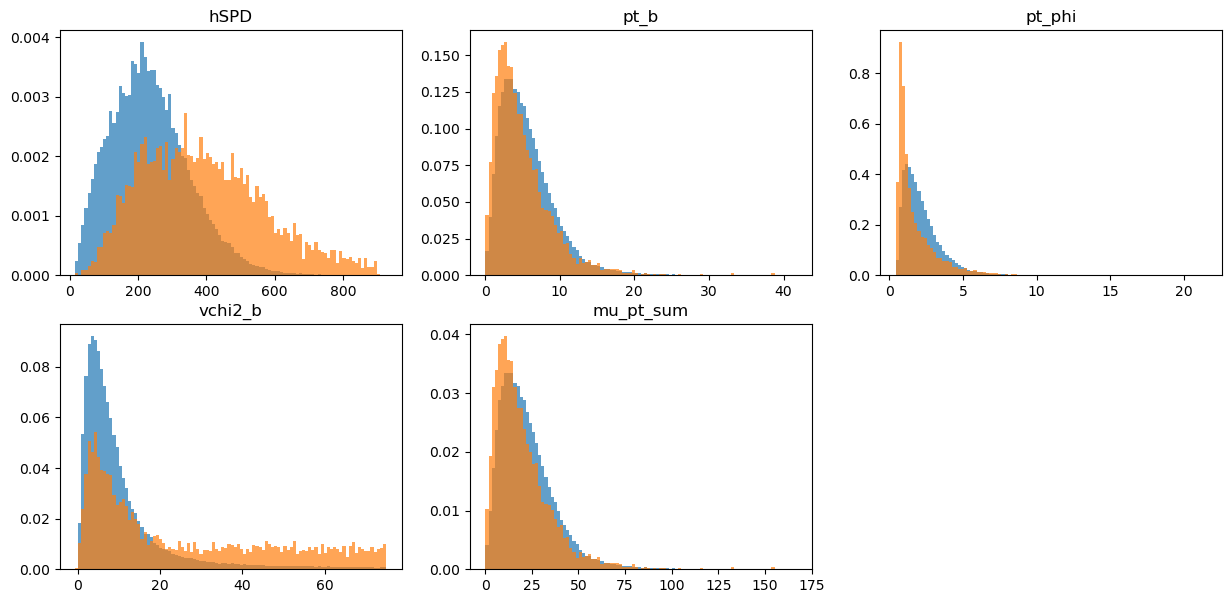This algorithm is inspired by gradient boosting and is able to fight curse of dimensionality. It uses decision trees and special loss functiion (ReweightLossFunction).

A classifier is trained to discriminate between real data and MC. This means we are able to reweight in several variables rather than just one. GBReweighter from hep_ml is able to handle many variables and requires less data (for the same performance).

GBReweighter supports negative weights (to reweight MC to splotted real data).

:

reweighter = reweight.GBReweighter(n_estimators=250, learning_rate=0.1, max_depth=3, min_samples_leaf=1000,
gb_args={'subsample': 0.4})
reweighter.fit(original_train, target_train)

gb_weights_test = reweighter.predict_weights(original_test)
# validate reweighting rule on the test part comparing 1d projections
draw_distributions(original_test, target_test, gb_weights_test)

KS over  hSPD  =  0.0347095621152409
KS over  pt_b  =  0.025211070013842152
KS over  pt_phi  =  0.03053187424458037
KS over  vchi2_b  =  0.03684974158016552
KS over  mu_pt_sum  =  0.025211070013842152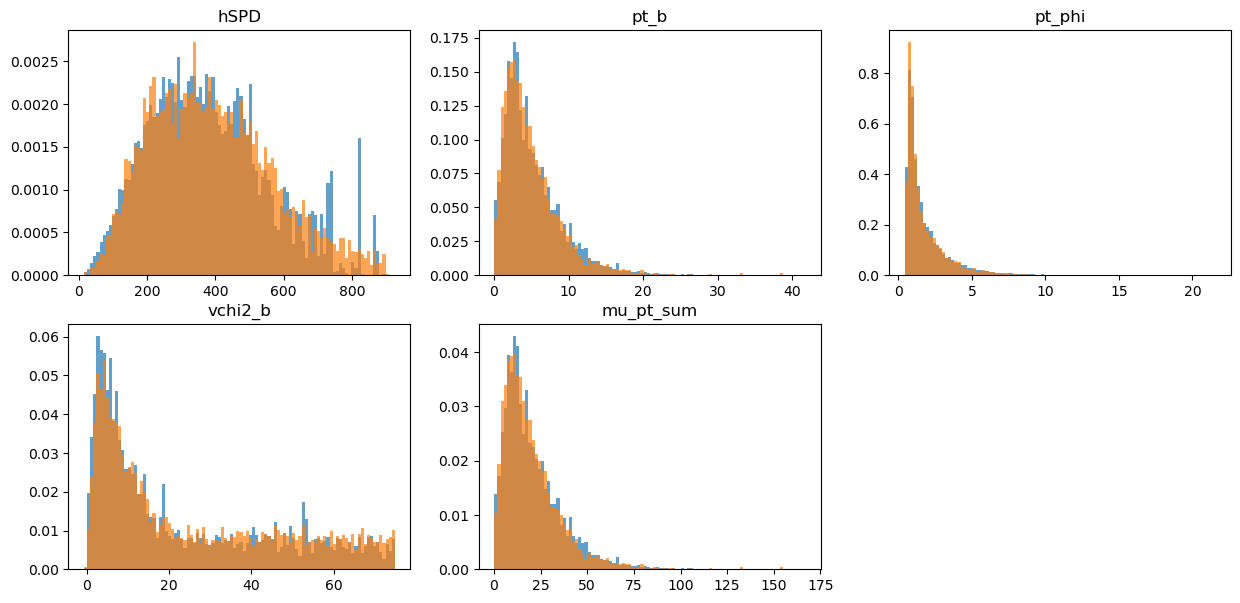### Comparing some simple expressions:

The most interesting is checking some other variables in multidimensional distributions (those are expressed via original variables). Here we can check the KS distance in multidimensional distributions. (The lower the value, the better agreement of distributions.)

:

def check_ks_of_expression(expression):
col_original = original_test.eval(expression, engine='python')
col_target = target_test.eval(expression, engine='python')
w_target = np.ones(len(col_target), dtype='float')
print('No reweight   KS:', ks_2samp_weighted(col_original, col_target,
weights1=original_weights_test, weights2=w_target))
print('Bins reweight KS:', ks_2samp_weighted(col_original, col_target,
weights1=bins_weights_test, weights2=w_target))
print('GB Reweight   KS:', ks_2samp_weighted(col_original, col_target,
weights1=gb_weights_test, weights2=w_target))

:

check_ks_of_expression('hSPD')

No reweight   KS: 0.5334475269539376
Bins reweight KS: 0.42863624879323187
GB Reweight   KS: 0.0347095621152409

:

check_ks_of_expression('hSPD * pt_phi')

No reweight   KS: 0.09012817049079563
Bins reweight KS: 0.11352328240467435
GB Reweight   KS: 0.02190268041831478

:

check_ks_of_expression('hSPD * pt_phi * vchi2_b')

No reweight   KS: 0.37901963253191406
Bins reweight KS: 0.34424900596261393
GB Reweight   KS: 0.031860685902892816

:

check_ks_of_expression('pt_b * pt_phi / hSPD ')

No reweight   KS: 0.4847105939189398
Bins reweight KS: 0.39303454488565615
GB Reweight   KS: 0.03632797862321624

:

check_ks_of_expression('hSPD * pt_b * vchi2_b / pt_phi')

No reweight   KS: 0.5001298186913166
Bins reweight KS: 0.4091388317915871
GB Reweight   KS: 0.013885328548535414


## GB-discrimination

Let’s check how well a classifier is able to distinguish these distributions.

For this puprose we split the data into train and test, then we train a classifier to distinguish between the real data and MC distributions.

We can use the ROC Area Under Curve as a measure of performance. If ROC AUC = 0.5 on the test sample, the distibutions are identical, if ROC AUC = 1.0, they are ideally separable. So we want a ROC AUC as close to 0.5 as possible to know that we cannot separate the reweighted distributions.

:

from sklearn.ensemble import GradientBoostingClassifier
from sklearn.metrics import roc_auc_score
from sklearn.model_selection import train_test_split

data = np.concatenate([original_test, target_test])
labels = np.array( * len(original_test) +  * len(target_test))

weights = {}
weights['original'] = original_weights_test
weights['bins'] = bins_weights_test
weights['gb_weights'] = gb_weights_test

for name, new_weights in weights.items():
W = np.concatenate([new_weights / new_weights.sum() * len(target_test),  * len(target_test)])
Xtr, Xts, Ytr, Yts, Wtr, Wts = train_test_split(data, labels, W, random_state=42, train_size=0.51)
clf = GradientBoostingClassifier(subsample=0.3, n_estimators=50).fit(Xtr, Ytr, sample_weight=Wtr)

print(name, roc_auc_score(Yts, clf.predict_proba(Xts)[:, 1], sample_weight=Wts))

original 0.9359999166274395
bins 0.9095742001748905
gb_weights 0.4893777931359195


### Great!

To the classifier, the two datasets seem now nearly undistingishable. Can we improve that? The GBReweighter is quite sensible to its hyperparameters, especially to n_estimators. Feel free to go back and increase it (to e.g. 250). What happens?

Answer: we get an even lower score! 0.49! Yeey! Or wait…

### What did just happen?

Our algorithm completely overfitted the distribution. The problem is that while for the classification we have a true label for each event, we do not have a true weight for each event, we just have a true distribution so a “true local density” of the events. Or something like that.

As powerful as the GBReweighter is, as much does it need some care to be taken when choosing the hyperparameters as it can easily overfit. And, worse, the overfitting cannot be spotted so easily.

While this is a topic on its own, whatever you do with the GBReweighter, be sure to really validate your result.

A hint of what may goes wrong is given when plotting the weights.

:

plt.hist(weights['gb_weights'], bins=50)
plt.yscale('log')
plt.title('predicted weights')

:

Text(0.5, 1.0, 'predicted weights')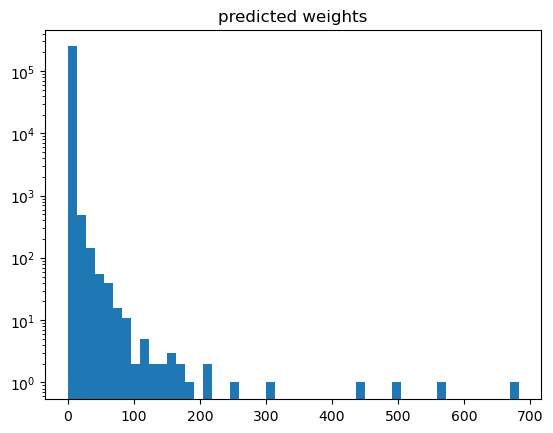:

np.max(weights['gb_weights']), np.sum(weights['gb_weights'])

:

(641.8825873922033, 69099.26440929338)


With such a high weight for a single event, this does not look desireable. And be aware of ad-hoc solutions: just clipping or removing weights is completely wrong as this would disturb the distribution completely.

A good way to proceed is to play around with the hyperparameters in order to avoid overfitting until the weights distribution looks “reasonable”. Especially we don’t want to have high weights in there if avoidable.

## How to tune

First find an appropriate number of estimators.

max_depth basically determines the order of correlations to be taken into account.

n_estimators has a tradeoff vs learning_rate. Increasing the former by a factor of n and reducing the latter by a factor of 1 / n keeps the reweighter with the same capability (e.g. overfitting) but tends to smooth out things. So use this at the end as more estimators take more time.

## Folding reweighter

FoldingReweighter uses k folding in order to obtain unbiased weights for the whole distribution.

The hyperparameters have been adjusted here. Be aware that n_estimators=80 with learning_rate=0.01 reads as n_estimators=8 and learning_rate=0.1 (in the above). So we greatly reduced the number of estimators.

:

# define base reweighter
reweighter_base = reweight.GBReweighter(n_estimators=80,
learning_rate=0.01, max_depth=4, min_samples_leaf=100,
gb_args={'subsample': 0.8})
reweighter = reweight.FoldingReweighter(reweighter_base, n_folds=2)
# it is not needed divide data into train/test parts; reweighter can be train on the whole samples
reweighter.fit(original, target)

# predict method provides unbiased weights prediction for the whole sample
# folding reweighter contains two reweighters, each is trained on one half of samples
# during predictions each reweighter predicts another half of samples not used in training
folding_weights = reweighter.predict_weights(original)

draw_distributions(original, target, folding_weights)

KFold prediction using folds column
KS over  hSPD  =  0.3069343038595975
KS over  pt_b  =  0.1808053376835848
KS over  pt_phi  =  0.3082330336192169
KS over  vchi2_b  =  0.2982631515629319
KS over  mu_pt_sum  =  0.1808053376835848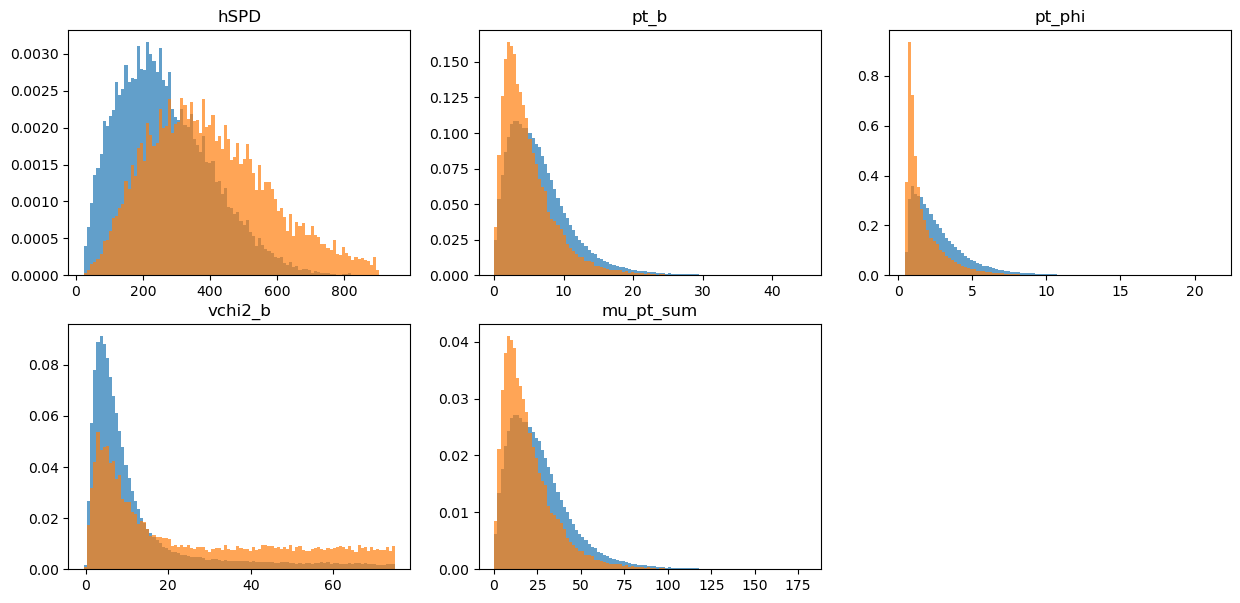## GB discrimination for reweighting rule

:

data = np.concatenate([original, target])
labels = np.array( * len(original) +  * len(target))

weights = {}
weights['original'] = original_weights
weights['2-folding'] = folding_weights

for name, new_weights in weights.items():
W = np.concatenate([new_weights / new_weights.sum() * len(target),  * len(target)])
Xtr, Xts, Ytr, Yts, Wtr, Wts = train_test_split(data, labels, W, random_state=42, train_size=0.51)
clf = GradientBoostingClassifier(subsample=0.6, n_estimators=30).fit(Xtr, Ytr, sample_weight=Wtr)

print(name, roc_auc_score(Yts, clf.predict_proba(Xts)[:, 1], sample_weight=Wts))

original 0.9369223085837554
2-folding 0.8268759949345327

:

plt.hist(weights['2-folding'], bins=50)
plt.yscale('log')
plt.title('predicted weights')

:

Text(0.5, 1.0, 'predicted weights')